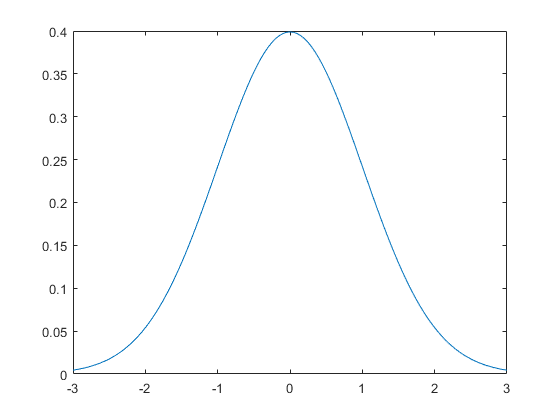# gaussian

Create a Gaussian distribution.

## Syntax

```gauss_distr = gaussian(x)
gauss_distr = gaussian(x, magnitude)
gauss_distr = gaussian(x, magnitude, mean)
gauss_distr = gaussian(x, magnitude, mean, variance)
```

## Description

`gaussian` returns a Gaussian distribution f(x) with the specified magnitude, mean, and variance. If these values are not specified, the magnitude is normalised and values of variance = 1 and mean = 0 are used. For example running

```x = -3:0.05:3;
plot(x, gaussian(x));
```

will plot a normalised Gaussian distribution.Note, the full width at half maximum of the resulting distribution can be calculated by `FWHM = 2 * sqrt(2 * log(2) * variance)`.

## Inputs

 `x` x-axis variable

## Optional Inputs

 `magnitude` bell height (default = normalised) `mean` mean or expected value (default = 0) `variance` variance ~ bell width (default = 1)

## Outputs

 `gauss_distr` Gaussian distribution

`getWin`